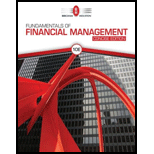# EXPECTED RETURN A stock’s returns have the following distribution: Demand For the Company’s Products Probability of this D-emand Occurring Rate of Return if this Demand Occurs Weak 0.1 (3%) Below average 0.1 (14) Average 0.3 11 Above average 0.3 20 Strong 0.2 45 1.0 Assume the risk-free rate is 2%. Calculate the stock’s expected return, standard deviation, coefficient of variation, and Sharpe ratio.### Fundamentals Of Financial Manageme...

10th Edition
Eugene F. Brigham + 1 other
Publisher: Cengage Learning
ISBN: 9781337902571

#### Solutions

Chapter
Section### Fundamentals Of Financial Manageme...

10th Edition
Eugene F. Brigham + 1 other
Publisher: Cengage Learning
ISBN: 9781337902571
Chapter 8, Problem 1P
Textbook Problem
50 views

## EXPECTED RETURN A stock’s returns have the following distribution: Demand For the Company’s Products Probability of this D-emand Occurring Rate of Return if this Demand Occurs Weak 0.1 (3%) Below average 0.1 (14) Average 0.3 11 Above average 0.3 20 Strong 0.2 45   1.0   Assume the risk-free rate is 2%. Calculate the stock’s expected return, standard deviation, coefficient of variation, and Sharpe ratio.

Summary Introduction

To determine: The stocks expected return, coefficient of variation, standard deviation, and Sharpe ratio.

Introduction:

The portfolio refers to a group of financial assets like bonds, stocks, and equivalents of cash. The portfolio is held by investors and financial users. A portfolio is constructed in accordance with the risk tolerance and the objectives of the company.

The expected return on stock refers to the anticipated profit or loss in the investor’s investment.

Standard deviation refers to the stand-alone risk associated with the securities. It measures how much a data is dispersed with its standard value. The Greek letter sigma represents the standard deviation.

The coefficient of variation is a tool to determine the investment’s volatility.

Sharpe ratio helps to determine the performance of the investment.

### Explanation of Solution

The formula to compute the expected return is as follows:

rp=i=1Nwiri=w1r1+w2r2+...+wNrN

Here

rp is the expected rate of return.

wi is the weight of the stock.

ri is the estimated rate of return.

N is the number of stocks.

The formula to compute the standard deviation is as follows:

σ=i=1N(rir)2Pi

Here

rp is the expected rate of return.

wi is the weight of the stock.

ri is the estimated rate of return.

N is the number of stocks.

σ is the standard deviation.

The formula to calculate the coefficient of variation is as follows:

Coefficientofvariation=Standarddeviation(σ)Expectedreturn(r)

The formula to calculate the Sharpe ratio is as follows:

Sharpe ratio=Expected returnRisk free rateσ

Here

σ is the standard deviation.

Compute expected return:

rp=i=1Nwiri=w1r1+w2r2+...+wNrNrp=[(0.1×(30%))+(0.1×(14%))+(0.3×11%)+(0.3×20%)+(0.2×45%)]=(3%)+(1

### Still sussing out bartleby?

Check out a sample textbook solution.

See a sample solution

#### The Solution to Your Study Problems

Bartleby provides explanations to thousands of textbook problems written by our experts, many with advanced degrees!

Get Started